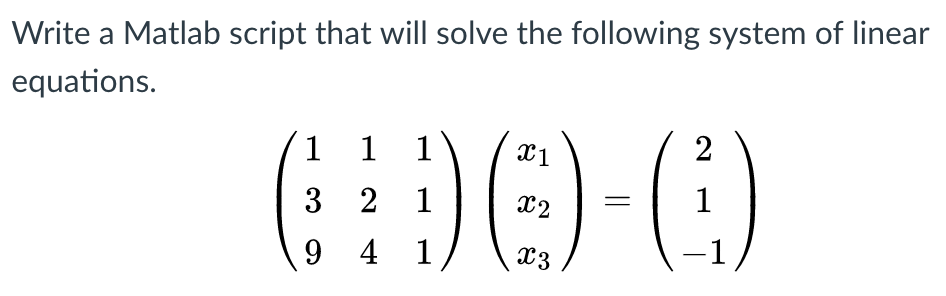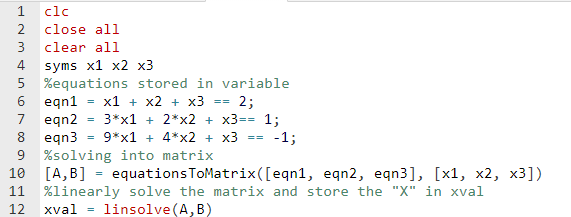# Question & Answer: Write a Matlab script that will solve the following system of linear equations. (1 1 1 3 2 1 9 4 1) (x_1 x_2 x_3) = (2 1 -1)…..Write a Matlab script that will solve the following system of linear equations. (1 1 1 3 2 1 9 4 1) (x_1 x_2 x_3) = (2 1 -1)

Don't use plagiarized sources. Get Your Custom Essay on
Question & Answer: Write a Matlab script that will solve the following system of linear equations. (1 1 1 3 2 1 9 4 1) (x_1 x_2 x_3) = (2 1 -1)…..
GET AN ESSAY WRITTEN FOR YOU FROM AS LOW AS \$13/PAGE

Solution:

Code:Copyable Code:

clc
close all
clear all
syms x1 x2 x3
%equations stored in variable
eqn1 = x1 + x2 + x3 == 2;
eqn2 = 3*x1 + 2*x2 + x3== 1;
eqn3 = 9*x1 + 4*x2 + x3 == -1;
%solving into matrix
[A,B] = equationsToMatrix([eqn1, eqn2, eqn3], [x1, x2, x3])
%linearly solve the matrix and store the “X” in xval
xval = linsolve(A,B)